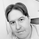Dilihat 1286 kali
V2: switched hardcoded timeframes and place were bulls and bears take controll
```study(title="[RS]Heiken Ashi Cloud V2", shorttitle="[RS]HACK.V2", overlay=true)
useAlternativeTF = input(false)
AlternativeTF = input("D")
TF = useAlternativeTF   ? AlternativeTF :
period == "1"   ? "30"  :
period == "3"   ? "60"  :
period == "5"   ? "120" :
period == "15"  ? "240" :
period == "30"  ? "480" :
period == "45"  ? "480" :
period == "60"  ? "720" :
period == "120" ? "720" :
period == "180" ? "D" :
period == "240" ? "D"   :
period == "D"   ? "W"   :
period == "W"   ? "M"   :
period == "M"   ? "3M"  : AlternativeTF

HA_ticker = heikenashi(tickerid)
HA_open = security(HA_ticker, TF, open)
HA_close = security(HA_ticker, TF, close)
HA_high = security(HA_ticker, TF, high)
HA_low = security(HA_ticker, TF, low)

HAO = plot(HA_open, color=maroon, linewidth=2)
HAC = plot(HA_close, color=green, linewidth=2)
HAH = plot(HA_high, color=silver, linewidth=1)
HAL = plot(HA_low, color=silver, linewidth=1)

fill(HAH,HAL,color=gray, transp=85)
fill(HAO,HAC,color=blue, transp=85)

//  ||---   Signals:
showLabels = input(true)
SEL0=cross(HA_open, HA_close) and HA_open >= HA_close
BUY0=cross(HA_open, HA_close) and HA_open <= HA_close

plotshape(not showLabels ? na : (SEL0 ? HA_high : na), style=shape.labeldown, color=maroon, location=location.absolute, textcolor=black, text='Bears\nIn Control')
plotshape(not showLabels ? na : (BUY0 ? HA_low : na), style=shape.labelup, color=green, location=location.absolute, textcolor=black, text='Bulls\nIn Control')

showBarColors = input(false)
BC_CON = HA_open < HA_close and BUY0 ? green : HA_open < HA_close ? lime : HA_open > HA_close and SEL0? maroon : HA_open > HA_close ? red : gray
barcolor(not showBarColors ? na : BC_CON)

```

## KomenThanks for the great indicators Ricardo. Finally profitable with your indicators!
This indicator is one of your best but I'm having the same problem as MrMister88. The labels appear where they weren't before, or appear double. Like two ted labels in a row or two green labels in a row.
Balaslynton99
@lynton99, its a repaint issue due to looking into a higher timeframe, for example the close of the daily on a intraday chart will change through out the day, but when you read close from previous day its allways the same value
Balas